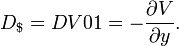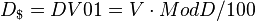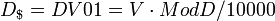#bonds #duration #finance #has-images

The dollar duration or DV01 is defined as negative of the derivative of the value with respect to yield:so that it is the product of the modified duration and the price (value):($per 1 percentage point change in yield) or($ per 1 basis point change in yield)
If you want to change selection, open document below and click on "Move attachment"

Bond duration - Wikipedia, the free encyclopedia
ar change in price for a $100 nominal bond for a one percentage point change in yield, is ($ per 1 percentage point change in yield) where the division by 100 is because modified duration is the percentage change. Dollar duration, DV01 <span>The dollar duration or DV01 is defined as negative of the derivative of the value with respect to yield: so that it is the product of the modified duration and the price (value): ($per 1 percentage point change in yield) or ($ per 1 basis point change in yield) The DV01 is analogous to the delta in derivative pricing (The Greeks) – it is the ratio of a price change in output (dollars) to unit change in input (a basis point of yield). Dollar dur